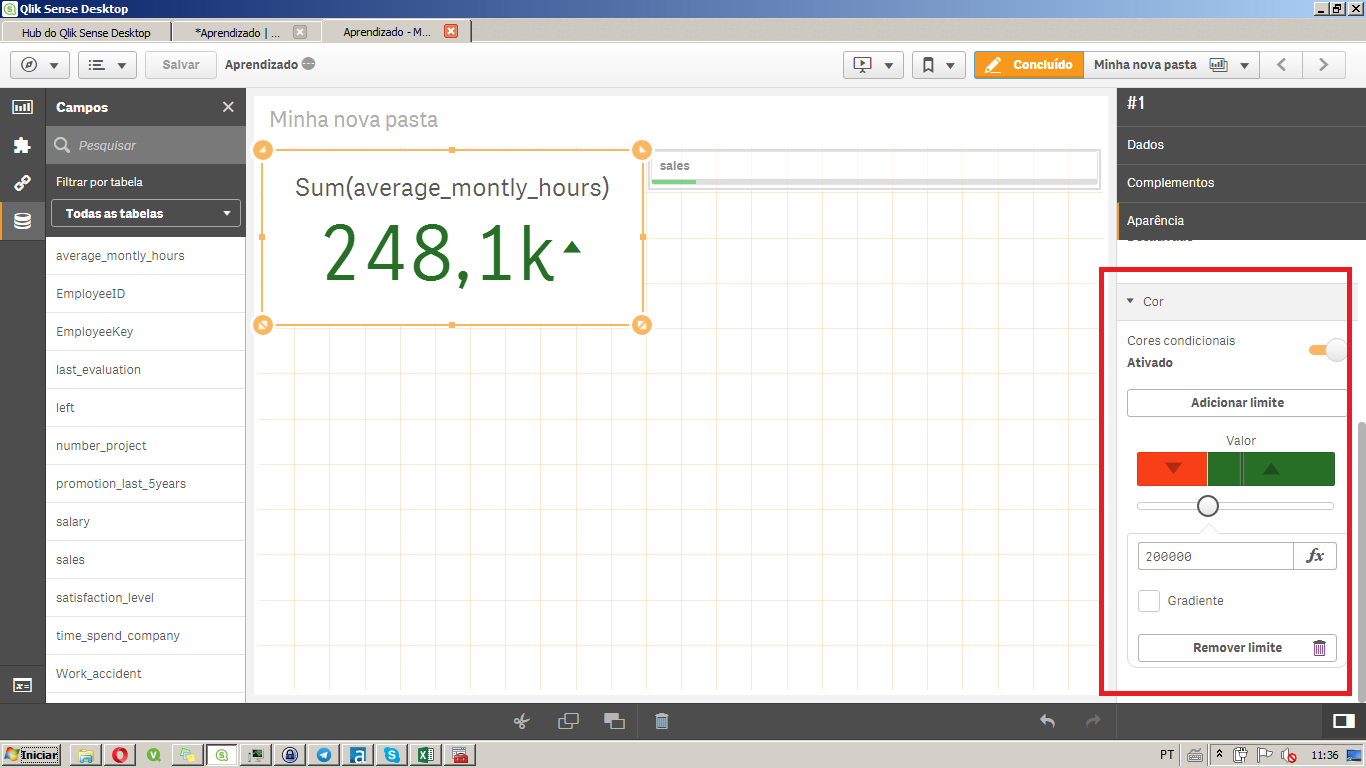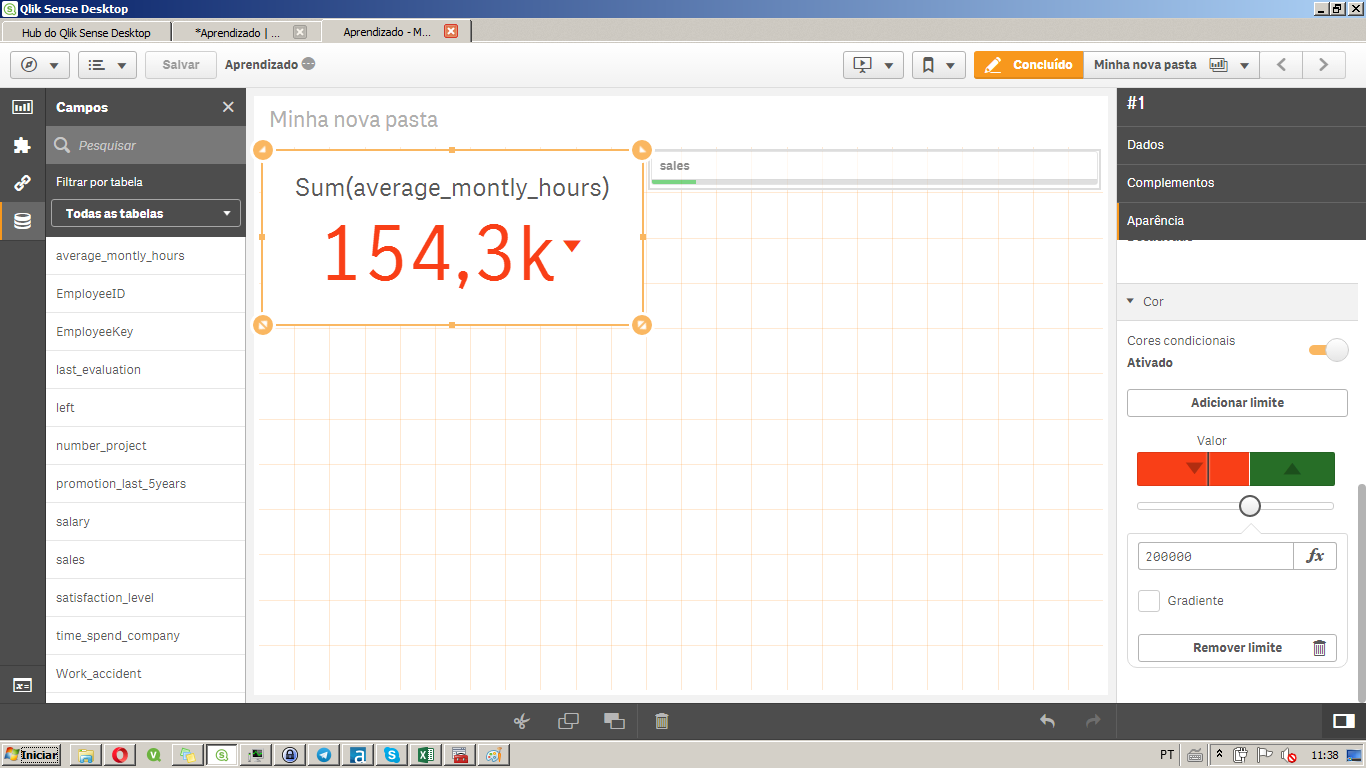New to Qlik Sense

Discussion board where members can get started with Qlik Sense.

Highlighted
Contributor II

Relative Text Color expression

Hi,

is it possible to write a relative text color expression? For example:

I would like if my expression is 5% below  target it to be red,

if it is 5% higher or lower than the Target it to be yellow,

and if it is 5% higher than it to be green.

is this possible? if so how would you write such an expression?

Thanks!

Tags (1)
1 Solution

Accepted Solutions
Valued Contributor II

Re: Relative Text Color expression

Were you able to change it to your need?

=If(Sum(Met_Goal_Flag)/Count(Met_Goal_Flag)>(Target*(1.05)), RGB(0,255,0),

If((Sum(Met_Goal_Flag)/Count(Met_Goal_Flag)>=(Target*(0.95)) and

(Sum(Met_Goal_Flag)/Count(Met_Goal_Flag)<=(Target*(1.05)))), RGB(255,255,0),

If(Sum(Met_Goal_Flag)/Count(Met_Goal_Flag)<(Target*(0.95)), RGB(255,0,0)

)

)

)

I hope brackets in this one are right.

10 RepliesPartner

Re: Relative Text Color expression

what's the qlik sense object are you talking about?Partner

Re: Relative Text Color expression

Hi erica,

If you mean the KPI object, there are the bellow options to do that (my limit is 200k, so above that green and up arrow, bellow that, red and down arrow).Contributor II

Re: Relative Text Color expression

The object is a chart, and I want to make the background of a field change colors

Valued Contributor II

Re: Relative Text Color expression

Hi Erica,

is it something similar to following thread

syntax to color text in a calculated column

Contributor II

Re: Relative Text Color expression

if(Sum(Met_Goal_Flag)/Count(Met_Goal_Flag)>(Target+(.05*Target)),'green', if(Sum(Met_Goal_Flag)/Count(Met_Goal_Flag)>(Target-(.05*Target)),'yellow','red'))

So I think I'm close, but it still isn't working properly. if the value is higher than the target it is returning red

Valued Contributor II

Re: Relative Text Color expression

try this

if(Sum(Met_Goal_Flag)/Count(Met_Goal_Flag)>(Target*(1.05)),'green',

if(Sum(Met_Goal_Flag)/Count(Met_Goal_Flag)>=(Target*(0.95))

and (Sum(Met_Goal_Flag)/Count(Met_Goal_Flag)<=(Target*(1.05)),'yellow',

'red'))

Contributor II

Re: Relative Text Color expression

Now only the yellow is working

Valued Contributor II

Re: Relative Text Color expression

Would you be able to provide me your sample data/app?

Valued Contributor II

Re: Relative Text Color expression

=If(Sum({<[Month/Year]={'8/1/2017'}>}COMM1) > 30, RGB(0,255,0),

If((Sum({<[Month/Year]={'8/1/2017'}>}COMM1) >= 10 and

(Sum({<[Month/Year]={'8/1/2017'}>}COMM1) <= 30)), RGB(255,255,0),

If(Sum({<[Month/Year]={'8/1/2017'}>}COMM1) < 10, RGB(255,0,0)

)

)

)It was bracketing issue